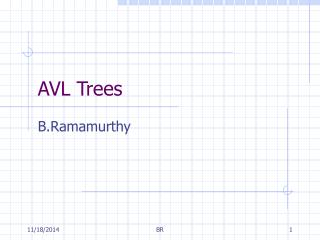DownloadDownload PresentationAVL Trees

# AVL Trees

Télécharger la présentation## AVL Trees

- - - - - - - - - - - - - - - - - - - - - - - - - - - E N D - - - - - - - - - - - - - - - - - - - - - - - - - - -
##### Presentation Transcript

1. AVL Trees B.Ramamurthy BR

2. Types of Trees binary search trees AVL trees 2-3 trees tries trees dynamic static game trees search trees priority queues and heaps graphs Huffman coding tree BR

3. Height of a Tree (Review) • Definition is same as level. Height of a tree is the length of the longest path from root to some leaf node. • Height of a empty tree is -1. • Height of a single node tree is 0. • Recursive definition: • height(t) = -1 if T is empty = 0 if number of nodes = 1 = 1+ max(height(LT), height(RT)) otherwise BR

4. Balanced Binary Search Trees • Let n be the number of nodes (keys) in a binary search tree and h be its height. • Binary search tree offers a O(h) insert and delete if the tree is balanced. • h is (log n) for a balanced tree. • Height-balanced(k) tree has for every node left subtree and right subtree differ in height at most by k. BR

5. Unbalanced Tree 45 54 65 Maximum height 78 87 98 BR

6. Balanced Tree 78 87 87 98 87 87 Minimum Height BR

7. AVL Property • AVL (Adelson, Velskii and Landis) tree is a height-balanced(1) tree that follows the property stated below: • For every internal node v of the tree, the heights of the children of v differ by at most 1. • AVL property maintains a logarithmic height in terms of the number of nodes of the tree thus achieving O(log n) for insert and delete. • Lets look at some examples. BR

8. Transforming into AVL Tree • Four different transformations are available called : rotations • Rotations: single right, single left, double right, double left • There is a close relationship between rotations and associative law of algebra. BR

9. Transformations • Single right : ((T1 T2) T3) = (T1 (T2 T3) • Single left : (T1 (T2 T3)) = ((T1 T2) T3) • Double right : ((T1 (T2 T3)) T4) = ((T1 T2) (T3 T4)) • Double left : (T1 ((T2 T3) T4)) = ((T1 T2) (T3 T4)) A A B B A B A B A B C A C B A B C A B C A,B,C are nodes, Tn (n =1,2,3,4..) are subtrees BR

10. X1 X2 X2 X3 X1 X3 X2 X3 AVL Balancing : Four Rotations X1 X3 Double right X2 X2 X1 Single right X3 X1 X1 X3 X2 Single left Double left X2 X1 X3 X1 X2 X3 BR

11. Example: AVL Tree for Airports • Consider inserting sequentially: ORY, JFK, BRU, DUS, ZRX, MEX, ORD, NRT, ARN, GLA, GCM • Build a binary-search tree • Build a AVL tree. BR

12. Binary Search Tree for Airport Names ORY ZRH JFK MEX BRU ORD DUS ARN NRT GLA GCM BR

13. ORY ORY JFK JFK BRU BRU An AVL Tree for Airport Names After insertion of ORY, JFK and BRU : Single right Not AVL balanced AVL Balanced BR

14. ORY JFK JFK BRU BRU ORY DUS MEX ZRH ORD NRT An AVL Tree for Airport Names (contd.) After insertion of DUS, ZRH, MEX and ORD After insertion of NRT? DUS MEX ZRH ORD Still AVL Balanced BR

15. JFK JFK Double Left ORY BRU BRU ORY DUS NRT ZRH DUS MEX ZRH MEX ORD ORD NRT An AVL Tree … Not AVL Balanaced Now add ARN and GLA; no need for rotations; Then add GCM BR

16. JFK ORY ORY DUS ARN BRU BRU NRT NRT ZRH ZRH GLA MEX ORD MEX ORD GCM An AVL Tree… JFK GCM ARN DUS GLA Double left NOT AVL BALANCED BR

17. For successful search, average number of comparisons: sum of all (path length to node+1) / number of nodes For the binary search tree (of airports) it is: 39/11 = 3.55 For the AVL tree (of airports) it is : 33/11 = 3.0 Search Operation BR